International
Tables for
Crystallography
Volume B
Reciprocal space
Edited by U. Shmueli

International Tables for Crystallography (2006). Vol. B, ch. 2.1, p. 190   | 1 | 2 |

## Section 2.1.1. Introduction

U. Shmuelia* and A. J. C. Wilsonb

aSchool of Chemistry, Tel Aviv University, Tel Aviv 69 978, Israel, and bSt John's College, Cambridge, England
Correspondence e-mail:  ushmueli@post.tau.ac.il

### 2.1.1. Introduction

| top | pdf |

The structure factor of thereflection is given by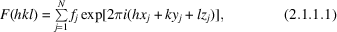whereis the atomic scattering factor [complex if there is appreciable dispersion; see Chapter 1.2and IT C (2004, Section 4.2.6)],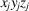are the fractional coordinates of the jth atom and N is the number of atoms in the unit cell. The present chapter is concerned with the statistical properties of the structure factor F and the intensity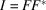, such as their average values, variances, higher moments and their probability density distributions.

Equation (2.1.1.1)expresses F as a function of two conceptually different sets of variables:taking on integral values in reciprocal space and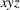in general having non-integral values in direct space, although the special positions tabulated for each space group in IT A (2005)may include the integers 0 and 1. In special positions, the non-integers often include rational fractions, but in general positions they are in principle irrational. Althoughandappear to be symmetrical variables in (2.1.1.1), these limitations on their values mean that one can consider two different sets of statistical properties. In the first we seek, for example, the average intensity of thereflection (indices fixed) as the positional parameters of the N atoms are distributed with equal probability over the continuous range 0–1. In the second, we seek, for example, the average intensity of the observable reflections (or of a subgroup of them having about the same value of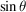) with the values ofheld constant at the values they have, or are postulated to have, in a crystal structure. Other examples are obtained by substituting the words probability density' for average intensity'. For brevity, we may call the statistics resulting from the first process fixed-index (continuously variable parameters being understood), and those resulting from the second process fixed-parameter (integral indices being understood). Theory based on the first process is (comparatively) easy; theory based on the second hardly exists, although there is a good deal of theory concerning the conditions under which the two processes will lead to the same result (Hauptman & Karle, 1953; Giacovazzo, 1977, 1980). Mathematically, of course, the condition is that the phase angle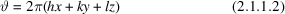should be distributed with uniform probability over the range 0–2π, whichever set of variables is regarded as fixed, but it is not clear when this distribution can be expected in practice for fixed-parameter averaging. The usual conclusion is that the uniform distribution will be realized if there are enough atoms, if the atomic coordinates do not approximate to rational fractions, if there are enough reflections and if stereochemical effects are negligible (Shmueli et al., 1984).

Obviously, the second process (fixed parameters, varying integral indices) corresponds to the observable reality, and various approximations to it have been attempted, in preference to assuming its equivalence with the first. For example, a third (approximate) method of averaging has been used (Wilson, 1949, 1981):are held fixed andare treated as continuous variables.

### References

International Tables for Crystallography (2005). Vol. A. Space-group symmetry, edited by Th. Hahn. Heidelberg: Springer.
International Tables for Crystallography (2004). Vol. C. Mathematical, physical and chemical tables, edited by E. Prince. Dordrecht: Kluwer Academic Publishers.
Giacovazzo, C. (1977). On different probabilistic approaches to quartet theory. Acta Cryst. A33, 50–54.
Giacovazzo, C. (1980). Direct methods in crystallography. London: Academic Press.
Hauptman, H. & Karle, J. (1953). Solution of the phase problem. I. The centrosymmetric crystal. Am. Crystallogr. Assoc. Monograph No. 3. Dayton, Ohio: Polycrystal Book Service.
Shmueli, U., Weiss, G. H., Kiefer, J. E. & Wilson, A. J. C. (1984). Exact random-walk models in crystallographic statistics. I. Space groupsand. Acta Cryst. A40, 651–660.
Wilson, A. J. C. (1949). The probability distribution of X-ray intensities. Acta Cryst. 2, 318–320.
Wilson, A. J. C. (1981). Can intensity statistics accommodate stereochemistry? Acta Cryst. A37, 808–810.